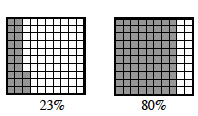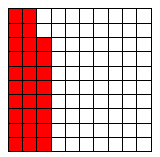### Home > CC1 > Chapter 3 > Lesson 3.1.3 > Problem3-50

3-50.

Use the Lesson 3.1.3B Resource Page   to shade in the amounts represented by the following descriptions, and then write them in the stated form.

1. Shade $7$ hundredths, and write the portion as a percent.

$7$ hundredths means you should shade $7$ boxes out of one hundred. Remember to write it as a percent. For help, refer to the information from the Math Notes box below.

Specific percents can be
appropriate number of squares.2. Shade 7 tenths, and write the portion as a fraction.

$7$ tenths is how many hundredths?

3. Shade 28%, and write the portion as a fraction.

The correct amount has been shaded for you. Remember to write the portion as a fraction.4. Shade 31.5%, and write the portion as a decimal.

The decimal is 0.315. Remember to shade 31.5%.

Use the eTool below to model each part.
Click the link at right for the full version of the eTool: 3-50 HW eTool